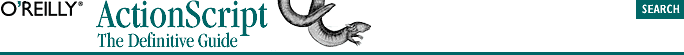home | O'Reilly's CD bookshelfs | FreeBSD | Linux | Cisco | Cisco Exam## 2.4. Types of Values

### 2.4.2. Automatic Value Conversion

In some contexts, ActionScript expects a specific type of data. If we use a variable whose value does not match the expected type, the interpreter attempts to convert the data. For example, if we use a text variable where a number is needed, the interpreter will try to convert the variable's text value to a numeric value for the sake of the current operation. In Example 2-2, z is set to 2. Why? Because the subtraction operator expects a number, so the value of y is converted from the string "4" to the number 4, which is subtracted from 6 (the value of x), yielding the result 2.

#### Example 2-2. Automatic String-to-Number Conversion

```x = 6;      // x is a number, 6
y = "4";    // y is a string, "4"
z = x - y;  // This sets z to the number 2```

Conversely, if we use a numeric variable where a string is expected, the interpreter attempts to convert the number to a string. In Example 2-3, z is set to the string "64", not the number 10. Why? Because the second operand in the expression x + y is a string. Therefore, the (+) performs string concatenation instead of mathematical addition. The value of x (6 ) is converted to the string "6" and then concatenated with the string "4" (the value of y), yielding the result "64".

#### Example 2-3. Automatic Number-to-String Conversion

```x = 6;      // x is a number, 6
y = "4";    // y is a string, "4"
z = x + y;  // This sets z to the string "64"```

Notice that the operator on line 3 (- in Example 2-2, + in Example 2-3), has a profound impact on the value assigned to z. In Example 2-2 the string "4" becomes the number 4, whereas in Example 2-3 the opposite occurs (the number 6 becomes the string "6"), because the rules for datatype conversion are different for the + operator than for the - operator. We'll cover data conversion rules in Chapter 3, "Data and Datatypes", and operators in Chapter 5, "Operators".

### 2.4.3. Determining the Type Manually

Automatic datatyping and conversion can be convenient, but as Example 2-2 and Example 2-3 illustrate, may also produce unexpected results. Before performing commands that operate on mixed datatypes, you may wish to determine a variable's datatype using the typeof operator:

```productName = "Macromedia Flash";  // String value
trace(typeof productName);         // Displays: "string"```

Once we know a variable's type, we can proceed conditionally. Here, for example, we check whether a variable is a number before proceeding:

```if (typeof age == "number"){
// okay to carry on
} else {
trace ("Age isn't a number");  // Display an error message
}```

For full details on the typeof operator, see Chapter 5, "Operators".